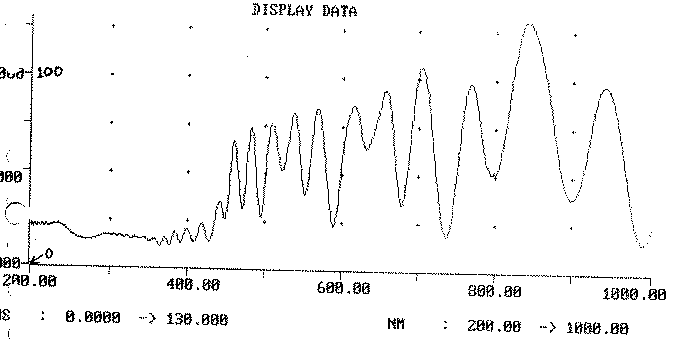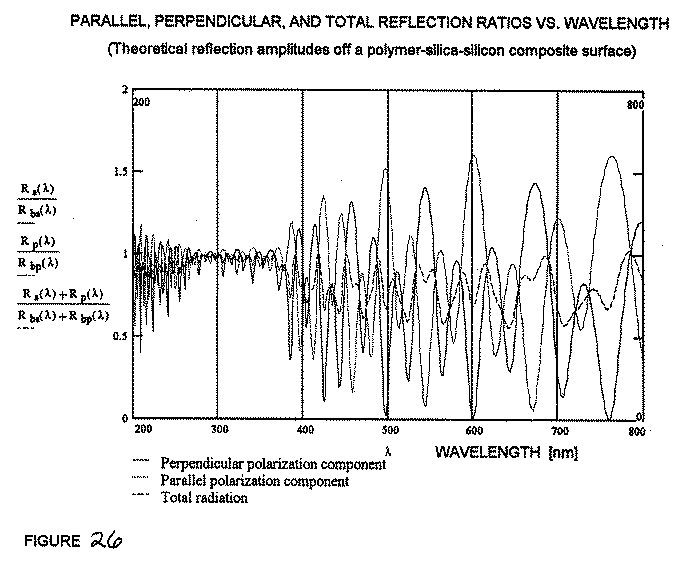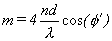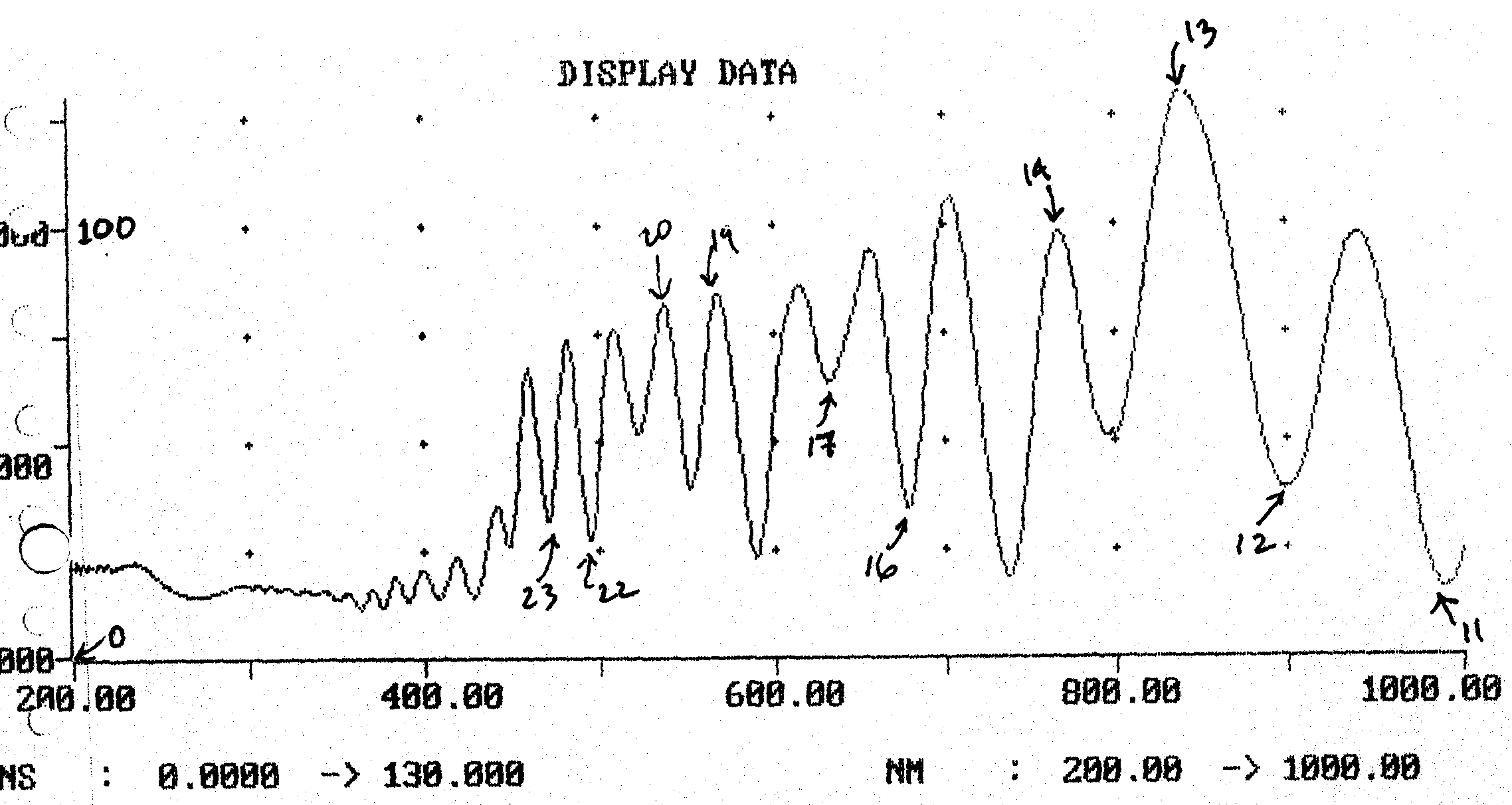﻿ Spectrometry and Ellipsometry Analysis -- Redeeming Time DesignsTHIN FILM ANALYSIS USING SPECTROMETRY AND ELLIPSOMETRY

OBJECTIVE 3:

III.  DOUBLE FILMS:

One of the oxidized wafers was analyzed in the ellipsometer for film thickness and index.  Then it was coated with a photoresist, a polymer used for lithography.  The wafer secured by vacuum to a spindle and spun while the photoresist liquid was applied.  The speed of the spindle increased afterward to increase film uniformity.  After spinning, the wafer was baked to harden the photoresist.  Then evaluation of this double film was attempted by all three methods.

III.1  NanoSpec:

The use of the NanoSpec is discussed first because it was the easiest.  The NanoSpec has a program to perform just such as analysis.  The double film wafer was just focused in the NanoSpec like any other wafer.  The program uses a value of n = 1.64.  The program returned a thickness value of 17,400 A.  Several points were taken but only this value will be given here.

III.2  Spectrophotometry:

The experimental spectrophotometry curve for the double film wafer is shown in Figure 25.  A MathCad program was written to simulate the condition in the film using, values from the NanoSpec.  Figure 26 shows the resulting plot.  There are similarities between the experimental curve and the parallel polarization component.  However, the resemblance is not as close as with the silica covered wafer.  One reason for this may be the fact that the "X" region of bare silicon was also coated with photoresist.  Therefore, the spectrophotometer may be observing two different conditions to some extent.FIGURE 25:FIGURE 26:  Parallel, Perpendicular, and Total Reflection Ratios versus Wavelength.  (Theoretical reflection amplitudes off a polymer-silica-silicon composite surface.)

No doubt, there are mathematical methods of determining film thickness and index from such a trace.  However, they would be too complicated to be used in this project.  Therefore, a simple method is used to determine n*d from the experimental plot.  This method uses the fact that there will be a maximum or minimum in the plot when the optical path length in the photoresist is equal to a whole number of wavelengths.  This is not true for the oxide layer due to the phase change that occurs at the substrate.  Fortunately, the value of photoresist thickness was determined by the NanoSpec.  Thus, using that value it is possible to check each peak to see if it is a peak for the photoresist.  This is accomplished by the following equation:16

where, a valid inflection point is found when m is a whole number and φ' is equal to 17.75 in this case.  A copy of the experimental data is shown on Figure 27, with m values written next to the valid peaks or valleys.  The data generated from this plot is presented in Table X.  A value of n of 1.64 was used in these calculations.FIGURE 27:

TABLE X

Data Points from a Spectrophotometer

Trace of Double Film on Silicon

 m λ [nm] d [nm] 23 468 17,230 22 494 17,400 20 536 17,160 19 568 17,270 17 632 17,200 16 679 17,390 14 765 17,140 13 838 17,440 12 898 17,250 11 992 17,470

Table X shows widely varying values of thickness.  They are not decreasing with wavelength, as might be expected since it was evaluated with constant n value.  Therefore, it is assumed that there is significant errors in the values.  Again this may be due to the "X" region.

III.3  Ellipsometry:

The ellipsometer is capable of determining both the n and d values for the top film if the properties of the lower film and the substrate are known.  The values of ψ and Δ returned for the double film are ψ = 33.24 and Δ = 71.76.  These values could now be put into a computer program and the values of n and d would be determined.  However, such a program was unavailable.  Therefore, the math to solve this problem will be presented.

See our Surface Oxide Tester, which  measures the film thickness' on copper in Angstroms with the speed and user-convenience required in QC Test Labs.